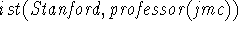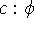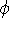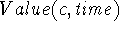Next: Lifting and Translation Up: Formalized context Previous: Formalized context

### Basic Properties of the Formal Theory of Context

The original proposals for context, [McC87b, McC93], have influenced a number of researchers to develop logics of context; these include [Sho91, Guh91, GSGF93, Nay94, AS95, BBM95, Buv96a]. We proceed with a short description of the logical properties of contexts and contextual reasoning.

1. Contexts are treated as formal objects, i.e. objects in the semantics which can be denoted by constants in the language and over which variables can range. Treating contexts as formal objects allows us to state relations between contexts in the same way we state relations between any other objects in the logic. For example, we can define a relation Specializes(c1,c2) which holds when context c2 is a specialization of context c1.

2. We introduce a new modality ist which is pronounced ``is true''. Formulas of the formare to be taken as assertions that the proposition p is true in context c. This allows us to express that, for example, John McCarthy is a professor in the context of Stanford University:. Note that sentences of the form ist(c,p) can themselves be true in contexts, e.g. we can have ist(c1,ist(c,p)). The ist modality also allows us to state lifting axioms--axioms relating what is true in one context based on what is true in another context. Lifting axioms are central for reasoning with multiple contexts; we will give examples of lifting axioms throughout the following sections.

3. All formulas are stated in some context, so we writewhen formulais given in context c. There is no outermost context; it is always possible to transcend the outermost context so far referred to. Transcendence is discussed in [McC93].

4. In order to capture the intuitive patterns of contextual reasoning, the formal system needs to contain rules for entering and exiting a context. For example, suppose we have the formula c0:ist(c,p). We can then enter the context c and infer the formula c:p. Conversely, if we have the formula c:p we can infer c0:ist(c,p) by exiting the context c.

5. Besides propositions being true in contexts, we also need the ability to talk about a value of a term t in context c, which we will write as Value(c,t). For example, we may need, when c is a context that has a time, e.g. a context usable for making assertions about a particular situation. In [MB94], we show how formulas containing the Value function can be treated as abbreviations of formulas containing ist.Next: Lifting and Translation Up: Formalized context Previous: Formalized context

Eyal Amir
Sat Mar 15 22:18:39 PST 1997